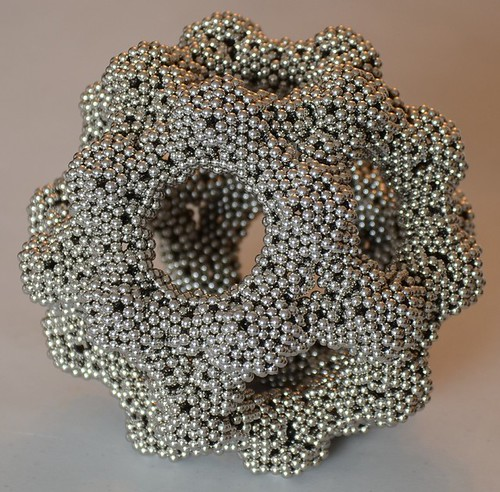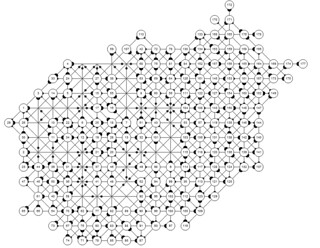## Morpion Solitaire GitHub

koozdra / Morpion (GitHub)

My library is now available. Here are some highlights:

Instantiation

`morpion = Morpion2::Morpion.new`

Random completion (from any state)

`morpion.random_completion`

Get the score:

`morpion.score`

Generating a dna array:

`dna = morpion.generate_dna`

Evaluating a dna array:

```eval_morpion = Morpion2::Morpion.new eval_morpion.eval_dna dna```

For a basic demo take a look at the demo folder for basic.rb. This code will perform a naive search and reset every minute.

Posted in Uncategorized | 3 Comments

## Grid Similarity

One way to compare two grids is by the positions of the made moves. Essentially we want to create a hash value to identify grids that have the same moves but could have different lines.

To demonstrate this effect I have put together a demonstration.

Use the left and right arrow keys to cycle between configurations.
Grids sharing points with different lines

Hashing

To achieve this we iterate over the taken moves on the grid. We form a collection of strings with their x and y values. The list is then sorted and joined together by a token.

`@taken_moves.collect{|move| "#{move.x},#{move.y}"}.sort.join('|')`

NOTE: To keep the string shorter I SHA1 encode the string.

This provides a very quick way to tell if this configuration of points has been seen after a dna modification.

Posted in Uncategorized | 1 Comment

## A Collection of High Scoring Grids in Pentasol Format

This is a collection of my highest scoring grids. There are some duplicates in there.

grids.zip

## Morpion DNA Encoding Revisited

Each move consists of two parts; a grid point where the move was made and a line that passes through the point.

Let’s consider the line universe of discourse. Each point on the grid can be the beginning of four possible lines. These possibilities per point encompass all the possible lines that can be formed.

Encoding

To store the possible lines for a 40×40 grid one would need an array of size 40x40x4.

The correspondence is defined by:

```def dna_line_index (line) line.x * 40 * 4 + line.y * 4 + DIRECTION_INDEX[line.dir] end```

The direction index is a consistent arbitrary ordering of the possible directions. I use:

```DIRECTION_INDEX = { :ne => 0, :e => 1, :se => 2, :s => 3 }```

Given a move’s line we can now get a corresponding value from the array.

The goal is to encode a completed grid into this array.

The array is initialized to a random real between 0 and 1 (needed for later).

`dna = Array.new(40*40*4){rand}`

We then run the following loop.

```@taken_moves.each_with_index do |move, index| dna[dna_line_index move.line] = (@taken_moves.length + 1) - index end```

Iterate over the taken moves of the grid and set the corresponding position in the array to a descending move numbering. For example if the completed grid has a score of 90 then the value stored in the array at the corresponding position of the first move’s line is 90. The second move’s line is 89 and so on.

Evaluation

The array can now be evaluated deterministically with the following method:

At each stage of the algorithm consider the set of possible moves. Rank each move using the value of the array index corresponding to the move’s line. Make that move. Continue the process until there are no possible moves.

This is a complete encoding. Evaluating the dna string will generate a consist grid.

Move Black Listing

The strength of this encoding is it’s resiliency to change. Consider the black listing of a move. A black listed move is a move that can only be made if it is the only possible move at an evaluation stage. This is accomplished quite easily with:

`dna[morpion.dna_line_index morpion.taken_moves.sample.line] = -1`

Since all the values in the array are positive this move will never be ranked higher than an available move. Also any undisturbed structures are preserved in their line preferences. A move that has been made will always be ranked higher than a move that was not made. If a situation occurs that all possible moves are not part of the original grid then a random move is taken. This is accomplished by initializing unused array indices to rand.

I find black listing three of the taken moves works really well.

related:

Coming soon…
How to efficiently identify two grids that have the same move positions (could have different lines)

End Search: Using loose moves, random completions and move index timeouts to generate similar solutions (hopefully better ones!)

Posted in Uncategorized | 8 Comments

## Two Dimensional Representations of the Penta Magnetic Constructor

The penta constructor is a very versatile constructor capable of many different configurations. The shape consists of 20 magnets.

Two Dimensional Representation:## High Resolution Fractal Backgrounds